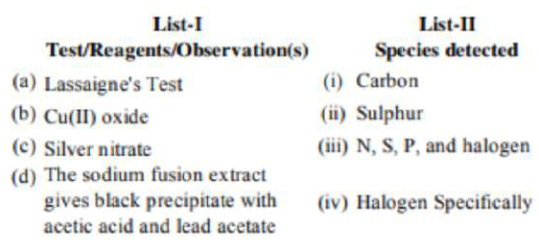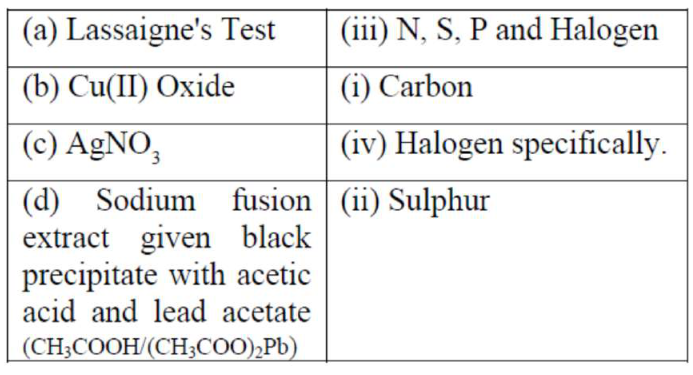# Match List-I with List-IIQuestion:

Match List-I with List-IIThe correct match is:

1. $(a)-(i i i),(b)-(i),(c)-(i i),(d)-(i v)$

2. (a) - (i), (b) - (iv), (c) - (iii), (d) - (ii)

3. (a) $-($ iii $),(b)-(i),(c)-(i v),(d)-(i i)$

4. (a) $-($ i $),($ b $)-($ ii $),($ c $)-($ iv $),($ d $)-($ iii $)$

Correct Option: , 3

Solution:

Match list:-Option-(a)-(iii) ; (b)-(i); (c)-(iv); (d)-(ii)